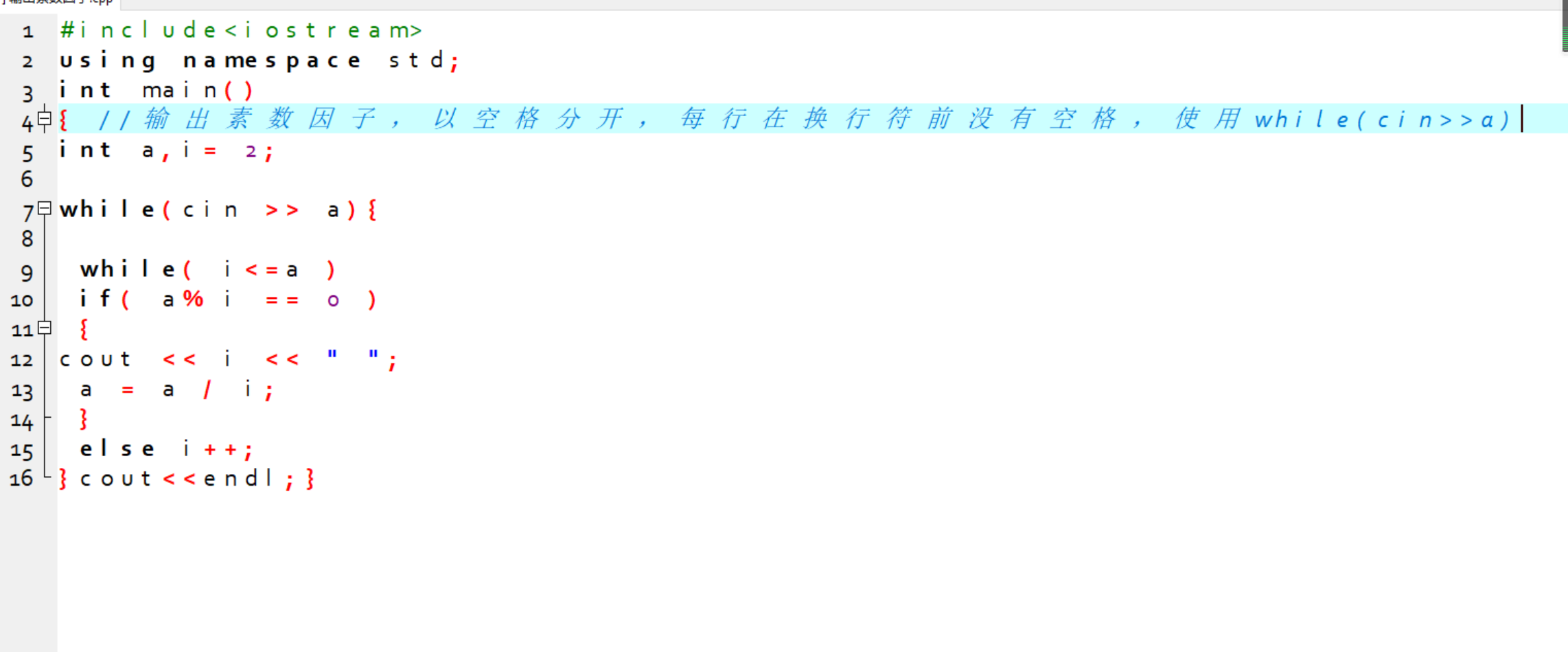2021-10-13 21:57

# c++输出一个整数的所有素数。• 好问题 提建议
• 收藏

#### 1条回答默认 最新

•qfl_sdu 2021-10-13 22:07
已采纳

代码如下：

``````#include <iostream>
using namespace std;
//判断素数
int isprime(int n)
{
int i = 2;
for(;i<n;i++)
{
if(n%i == 0)
return 0;
}
if(i==n) return 1;
return 0;
}

int main()
{
int i,n,flag;
while(cin >> n)
{
flag = 0;
for(i = 2;i<=n;i++)
{
if( (n%i == 0) && isprime(i))//是因子，并且是素数
{
if(flag == 0)
{
flag = 1;
cout << i;
}else
cout << " "<< i;
}
}
if(flag == 0)
cout << "该数没有素数因子"<<endl;
else
cout << endl;
}
return 0;
}

``````
已采纳该答案
评论
解决 无用
打赏 举报